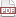### Raking to complete missing data

08 December 12. [link]PDF version

In this episode, we start with a data set with some complete observations and some observations with certain dimensions missing, and generate a PMF for the values of the complete data that accommodates all of the information in the partially-observed observations.

Further, we're going to do this using the same off-the-shelf raking routine as used in the last few episodes to adjust one data set toward another and to generate synthetic data.

Last timeLast time, when I showed you how we can synthesize data via raking, I presented a little trick to specify the table of margins, by including margins with missing values (represented by the IEEE NaN marker). If I have this data--

 X Y Z count NaN 2 3 10 1 2 3 10 1 NaN 3 10

--then the (Y, Z) margin of (2, 3) has total weight of 20 on it, as does the (X, Z) margin of (1, 3) . For synthesis, the trick of specifying NaNs but summing to not-NaN margins let me specify margins directly, rather than thinking about how I could invent a data set that happens to have the right margins.

This time, we'll read the NaNs as missing data. Having NaNs mixed in all over like this is formally known as a non-monotone missingness pattern, but I prefer the term Swiss cheese data. Simply summing the observations to margins produces a set of margins that includes the observed information, including what information is embodied in partially observed data. But we don't just want margins, we want the probabilities for specific cells.

We can use the complete data as a starting point, and rake to find the closest data set consistent with the complete information in the margins. The final result can be proven to have some desirable properties; see Little & Rubin for details (Amazon page). They view the problem as a Bayesian updating problem, using the information in the partially-observed observations to update the distribution of the fully-observed cells, and they arrive at the same raking algorithm here. Note, by the way, that their text uses only individual contrasts (in the notation here, .contrasts=(char *[]){"X", "Y", "Z"}) but nothing keeps us from using higher margins as well, like .contrasts=(char *[]){"X|Y", "X|Z", "X|Z"}. Doing so would allow us to retain more cross-dimension information than their treatment.

Here's the cut-n-pasteable sample code. It's longer than before (and more Apophenia-specific) because I added a little function to make draws from the resulting completed table. That function estimates a model from the data set, via apop_estimate(d, apop_pmf), then draws rows of data from the PMF. The rows get written to a new data set that the function allocates and fills.

Also, there's a weighting step, currently commented out, which I'll discuss below.

apop_text_to_db -O  -d="|" '-' cheese nans.db <<"----------"
row | col | weight
1 |  1 |   5
1 |  2 |   5
2 |  1 |   5
2 |  2 |   5
1 |  nan |   5
2 |  nan |   15
nan |  1 |   15
nan |  2 |   5
----------

cat <<"----------" > rake.c
#include <apop.h>

apop_data* make_draws(apop_data *d, int count, gsl_rng *r){
apop_data *draws=apop_data_alloc(count, d->matrix->size2);
apop_model *m = apop_estimate(d, apop_pmf);
for (int i=0; i< count; i++){
Apop_row(draws, i, onerow);
apop_draw(onerow->data, r, m);
}
apop_data_show(draws);
return draws;
}

int main(){
apop_db_open("nans.db");
apop_table_exists("init", 'd');
apop_query("create table init as select * from cheese "
"where row + col is not null");
apop_data *filledin= apop_rake(.margin_table="cheese", .count_col="weight",
.contrasts=(char*[]){"row", "col"}, .contrast_ct=2,
.init_table="init", .init_count_col="weight",);
//gsl_vector_scale(filledin->weights, 1./apop_vector_sum(filledin->weights));
apop_data_show(filledin);

gsl_rng *r = apop_rng_alloc(342134);
make_draws(filledin, 20, r);
}
----------

export CFLAGS="-g -Wall -O3  pkg-config --cflags apophenia"
export LDLIBS="pkg-config --libs apophenia" CC=c99
make -s rake && ./rake


One detail about the setup above: the totals might not be right. Say that our contrasts were Z and X| Y ; then the first contrast has a total not-NaN weight of 30, and the second has a total not-NaN weight of 10. The raking algorithm would thus rescale the table to sum to weight 30, then rescale to sum to weight 10, back and forth ad nauseum. The test for convergence only occurs at the end of a full round of raking, so the algorithm can still halt, but the total weight in the table will be determined by that last contrast. In this case, we'd have a total weight of ten; if we had ordered the contrasts to be X| Y then Z , we'd have weight 30. One option would be to add a reweighting step at the end of the sample code above, which I left in as a comment. It's unnecessary here because if the data gets treated as a PMF, the total weight of the vector of weights doesn't matter anyway. For other uses, you might want the scaling explicitly done.